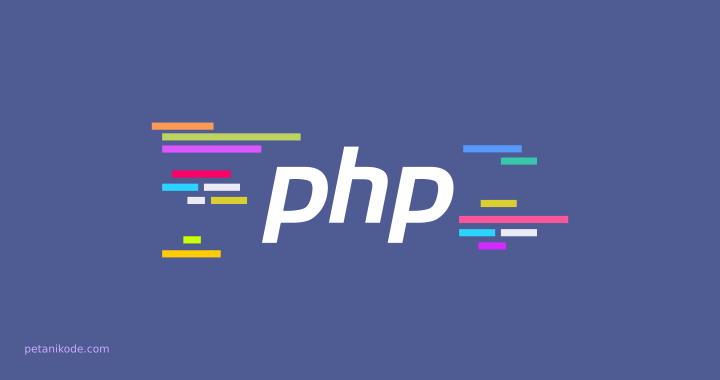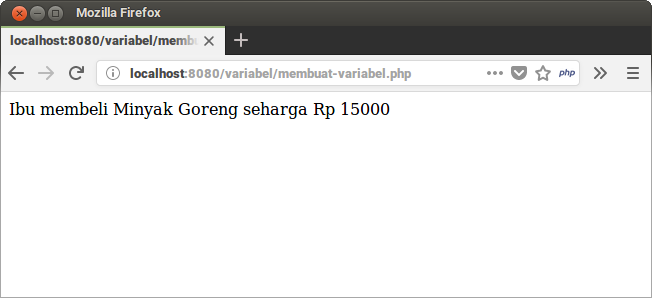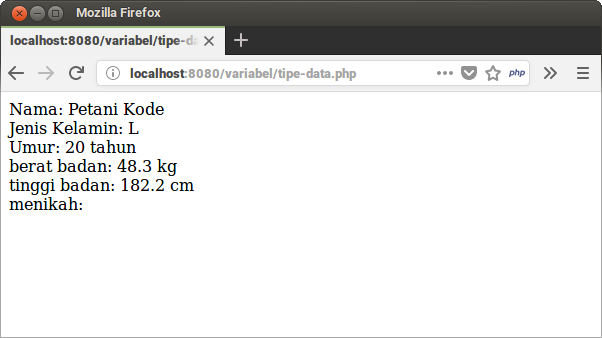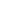| lesson material | material summary | questions and answers | definitions | types and examples | other information | materi pelajaran | ringkasan materi | pertanyaan dan jawaban | definisi | jenis-jenis dan contoh-contoh | informasi lainnya |

# Learning PHP: 5 Basic Things You Need to Know about Variables and Data TypesHave you ever discovered `x`and been `y`in a mathematics lesson?
Both of them are actually variables that store things.
Sometimes, we are often given the task to find out the contents of `x`and `y`.
Example:
``````jika x + 3 = 5, Berapakah x?
``````
Variables in programming also have the same meaning as in mathematics.
Variables are places where we store temporary values.
Variables will exist during the program. But we can also delete it from memory.
Later we will learn how.
1. How to create variables and store values ​​there
2. How to take values ​​from variables
3. Identify what data types can be stored in variables
4. Data type conversion
5. Remove variables from memory
Let's start…

## 1. Creating Variables in PHP

In PHP, we create a variable with a dollar sign ( `\$`), then followed by the name of the variable and the value we want to save.
Example:
``````<?php

\$harga = 1000;
``````
We just created a variable named `\$harga`with contents `1000`.
A sign equal to ( `=`) is a symbol or operator used to fill values ​​into variables.
Easy isn't it?
Let's try another example:
``````<?php

\$nama_barang = "Kopi C++";
\$harga = 4000;
\$stok = 40;
``````
Anyway, variables can also be refilled with new values.
Example:
``````<?php

// membuat variabel baru
\$stok = 40;

// mengisi ulang variabel dengan nilai baru
\$stok = 34;
``````
In making variable names there are several things that must be considered:
• The beginning of the variable name cannot use numbers and symbols, except the underscore.
Example:False
``````\$!nama = "";
\$46rosi = "";
``````
Example:Right
``````\$nama = "";
\$rosi46 = "";
``````
• Variable names consisting of two syllables, can be separated from the underscore ( `_`) or use the camelCase style .
Example:
``````\$nama_barang = "Buku PHP";
\$namaPembeli = "Petani Kode"; // <-- menggunakan camelCase
``````
• Variables must be filled when they are created. If we don't want to fill in, just fill in the blank value.
Example:
``````\$nama_barang = "";
\$namaPembeli = "";
\$harga = 0;
``````
• Variable names are Case Sensitive , meaning uppercase and lowercase letters are distinguished.
Example: These three variables will be considered different.
``````\$Belajar = "";
\$BELAJAR = "";
\$belajar = "";
``````

## 2. Taking Values ​​from Variables

After we create a variable, we will usually use it in the next process by taking the value.
Yes yes, cooking is only made and not used.
We can do values ​​from variables by writing their names in commands `echo`and other expressions.
Example:
``````<?php

// membuat variabel baru
\$nama_barang = "Minyak Goreng";
\$harga = 15000;

// menampilkan isi variabel
echo "Ibu membeli \$nama_barang seharga Rp \$harga";
``````
The result:If we use double quotes ( `"`) inside `echo`, then we can write the variable name directly like this:
``````\$judul = "Belajar PHP dari nol sampai expert";
echo "Judul artikel: \$judul";
``````
But ...
If we use single quotes ( `'`), then we must use a point to combine text with the variable.
Example:
``````\$judul = "Tutorial PHP untuk Pemula";
echo 'Judul artikel: '.\$judul;
``````
The dot on the command `echo`functions to combine the text in the variable with the text that we are going to print.

## 3. Get to know Data Types in PHP

The variables that we have created can we save with various types of data.
These data types are called data types.
There are several types of data types that can be stored in variables:
1. Char data type (character)
2. String data type (text)
3. Integer data type (number)
4. Float data type (fraction)
5. Boolean data type
6. Object data type
7. Array data type
8. NULL
9. etc.
PHP PHP, we don't have to declare the data type explicitly. Because PHP has been able to recognize data types from the values ​​we provide.
Example:
``````<?php

// tipe data char (karakter)
\$jenis_kelamin = 'L';

// tipe data string (teks)
\$nama_lengkap = "Petani Kode";

// tipe data integer
\$umur = 20;

// tipe data float
\$berat = 48.3;

// tipe data float
\$tinggi = 182.2;

// tipe data boolean
\$menikah = false;

echo "Nama: \$nama_lengkap<br>";
echo "Jenis Kelamin: \$jenis_kelamin<br>";
echo "Umur: \$umur tahun<br>";
echo "menikah: \$menikah";
``````
The result:The variable `\$menikah`will appear blank, because the value `false`will be converted to blank in the string.
Later we will discuss how to convert data types in PHP.
Now let's discuss each data type in more detail ...

### Char Data Types and Strings in PHP

Char is a data type consisting of characters. The writing is enclosed in quotation marks one.
Example:
``````\$huruf = 'E';
``````
Then, a String is a data type consisting of a collection of characters. The writing is enclosed in double quotation marks.
Example:
``````\$alamat = "Jl. Mawar, Jakarta";
``````

### Integer Data Types in PHP

Integer is a numeric data type. The writing does not use quotation marks.
Example:
``````\$nilai = 98; // angka positif
\$poin = -31; // angka negatif
``````

### Float Data Type in PHP

Float is a fraction data type. Just like integers, this data type is written without quotes.
Example:
``````\$panjang = 233.12;
\$kapasistas = 13232.12;
``````
Also the float data type is written in a notation like this:
``````\$jarak = 1.2E-5;
``````
`E-5`meaning the exponent of `10`.
The above example will be the same as If we describe it will become .`1.2 x 10-5``0.000012`
In order for the float format not to be printed in the notation `E`, we can use the function `sprintf()`.
Example:
``````echo sprintf('%f', \$a);
// batasi angka di belakang koma
echo sprintf('%.3f', \$a);
``````

### Boolean data type in PHP

Boolean data types are data types that are only valuable `ture`and `false`.
Writing `true`and `false`not enclosed with quotation marks.
Example:
``````\$isActive = false;
\$menikah = true;
``````

### Array Data Types in PHP

An array is a data type that contains a set of data.
Example:
``````\$minuman = array("Kopi", "Teh", "Jus Jeruk");
\$makanan = ["Nasi Goreng", "Soto", "Bubur"];
``````
We will discuss more about arrays in: 7 Things You Need to Know About Arrays .

### Object Data Types in PHP

Object data types are abstract data types that contain data and methods .
Example:
``````\$user = new User();
``````
Object data types are more often called instances of a class. In the example above, `User()`the class is instance variable `\$user`.
Every instance of creation must be followed by keywords `new`.

## NULL Data Type in PHP

Data type `NULL`is a data type that declares blank.
Meaning: If we fill a variable with a value `NULL`, then the variable will be considered empty or has no value.
Example:
``````\$nama = NULL;
``````

## 4. Conversion Data types in PHP

What will happen if we do division with text data types with numbers like this:
``````\$a = 3;
\$b = "angka 10";

\$c = \$b / \$a;
``````
Of course there will be an error when we want to print the contents of the variable `\$c`.
Therefore, we must first convert the data type so that another operation can be carried out.
In PHP data type conversion can be done by operator kali ( `*`).
Example:
``````<?php
\$foo = "1";  // mula-mual \$foo dalam bentuk string (ASCII 49)
\$foo *= 2;   // \$foo sekarang adalah integer (2)
\$foo = \$foo * 1.3;  // \$foo sekarang adalah float (2.6)
\$foo = 5 * "10 Little Piggies"; // \$foo sekarang adalah integer (50)
\$foo = 5 * "10 Small Pigs";     // \$foo sekarang adalah integer (50)
?>
``````
Besides this method, we can also do it like in C:
``````<?php

\$a = "32";
\$a = (int) \$a; // ubah nilai a menjadi integer
\$a = (float) \$a; // ubah nilai a menjadi float
\$a = (string) \$a; // ubah nilai a menjadi string

?>
``````

## 5. Removing Variables from Memory

If there are variables that are no longer needed, then we can delete them to improve program performance.
How to delete variables in PHP can use functions `unset()`.
Example:
``````// membuat variabel \$tmp
\$tmp = 2901;

// menghapus variabel \$tmp
unset(\$tmp);

// mencoba mengakses variabel \$tmp
echo \$tmp;
``````
If we execute the code above, an error will occur:
``````PHP Notice:  Undefined variable: tmp
``````
Because `\$tmp`we deleted the variable .
Why must delete the variable? later it will also be deleted when the program is finished?
Usually when we want optimization, unused variables must be removed so that they don't overload the memory on the server.

##### 0 Komentar untuk "Learning PHP: 5 Basic Things You Need to Know about Variables and Data Types"

Silahkan berkomentar sesuai artikel

Template By Kunci Dunia